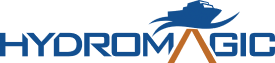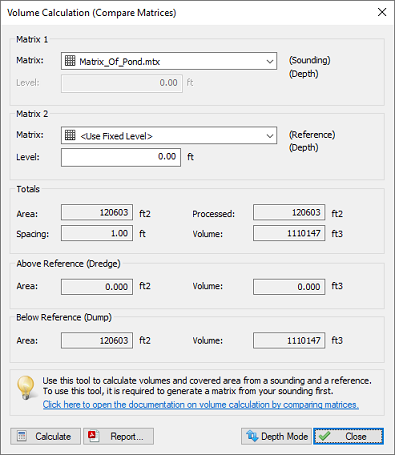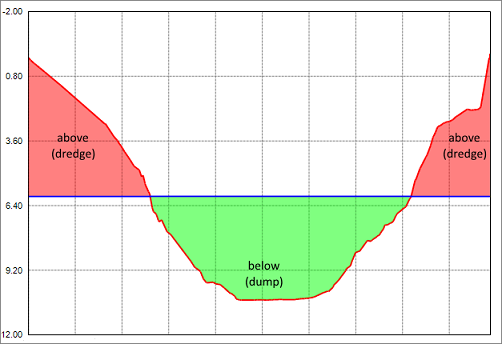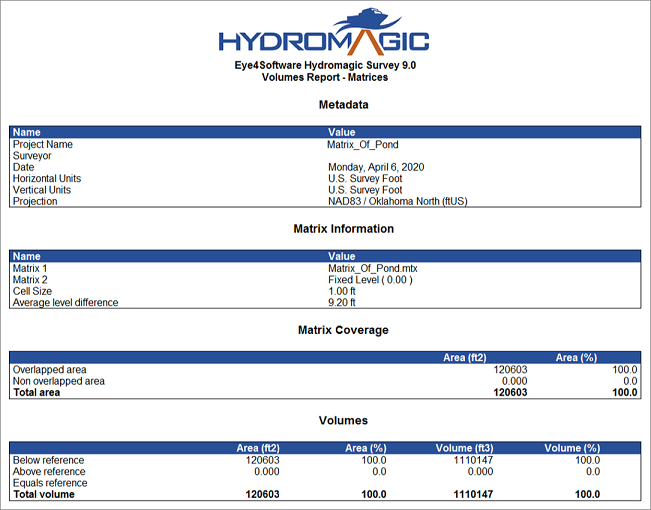## Hydrographic Survey Software

### Volume calculation using matrix

The matrix volume calculation tool can be used in two ways: to calculate the difference in volume between two matrices (for example: pre-dredge and post-dredge), or between a matrix and a fixed level. The latter option can be used to calculate the area and volume of a pond or basin or tank (you can also use staging volume calculations for this).

To use this option for volume calculation, you must first have generated a matrix from your corrected sounding(s). This tool calculates volumes by multiplying the difference between depth in the selected matrices with the dimensions of a single matrix cell. When comparing matrices, make sure that they are both generated with the same matrix spacing (cell size) setting.

To start the matrix volume calculation tool, select the "Matrix Volumes..." option from the "Tools" => "Volume Calculations" menu. The following dialog will be shown:Select two matrices or one matrix and a fixed level to calculate volumes.

### Volume calculation conventions

In Hydromagic, the following conventions are used when calculating volumes:

Sounding data above the fixed reference or channel design is used to calculate the "above volume". This is normally the amount of material that has to be dredged in order to get the channel design. Sounding data below the fixed reference or channel design is used to calculate the "below volume". This is normally the amount of material that has to be dumped in order to get the channel design.

In "depth mode" a value higher then the reference value is considered below. Please note that in "elevation mode", when a vertical reference (datum) is used, it is the other way around. In this case values less then the reference value are used to calculate the below values.

The following image shows an example of a volume calculation (in "depth mode") with above and below values:This image shows the difference between "above" and "below" volume values.

### Selecting Matrices

#### Matrix 1

Use this setting to specify the matrix generated from your sounding, or when using two matrices, the matrix generated from the post-dredge sounding.

#### Matrix 2

Use this setting to specify the matrix generated from your pre-dredge sounding or a matrix generated from a 3D CAD file or channel design. In case you are calculate the volume of a basin or pond, select the "<Use Fixed Level>" option, and enter the level of the water surface. When no tide correction is used, this value is 0.00.

### Calculate Volumes

Click the "Calculate" button to calculate areas and volumes for the selected matrices. Calculated values are split in totals, above reference (Matrix 2) and below reference (Matrix 2).

#### Totals

This section will display the total area and volume for the whole sounding. The processed area means the amount of overlap between the two matrices used. When a fixed level is used, the overlap is always 100% and the processed area will be the same as the total area.

#### Below Reference

Area and volume below the level found in Matrix 2 (see volume calculation conventions paragraph)

#### Above Reference

Area and volume above the level found in Matrix 2 (see volume calculation conventions paragraph)

### Report

Click the "Report" button to calculate area's and volumes from the selected matrices. This function creates a PDF report containing the calculated areas and volumes. Please note that PDF reader software needs to be installed on your computer. In Windows 10 you can use the built in "Reader" application.Click the "Report..." button to generate a PDF report for the volume calculations.